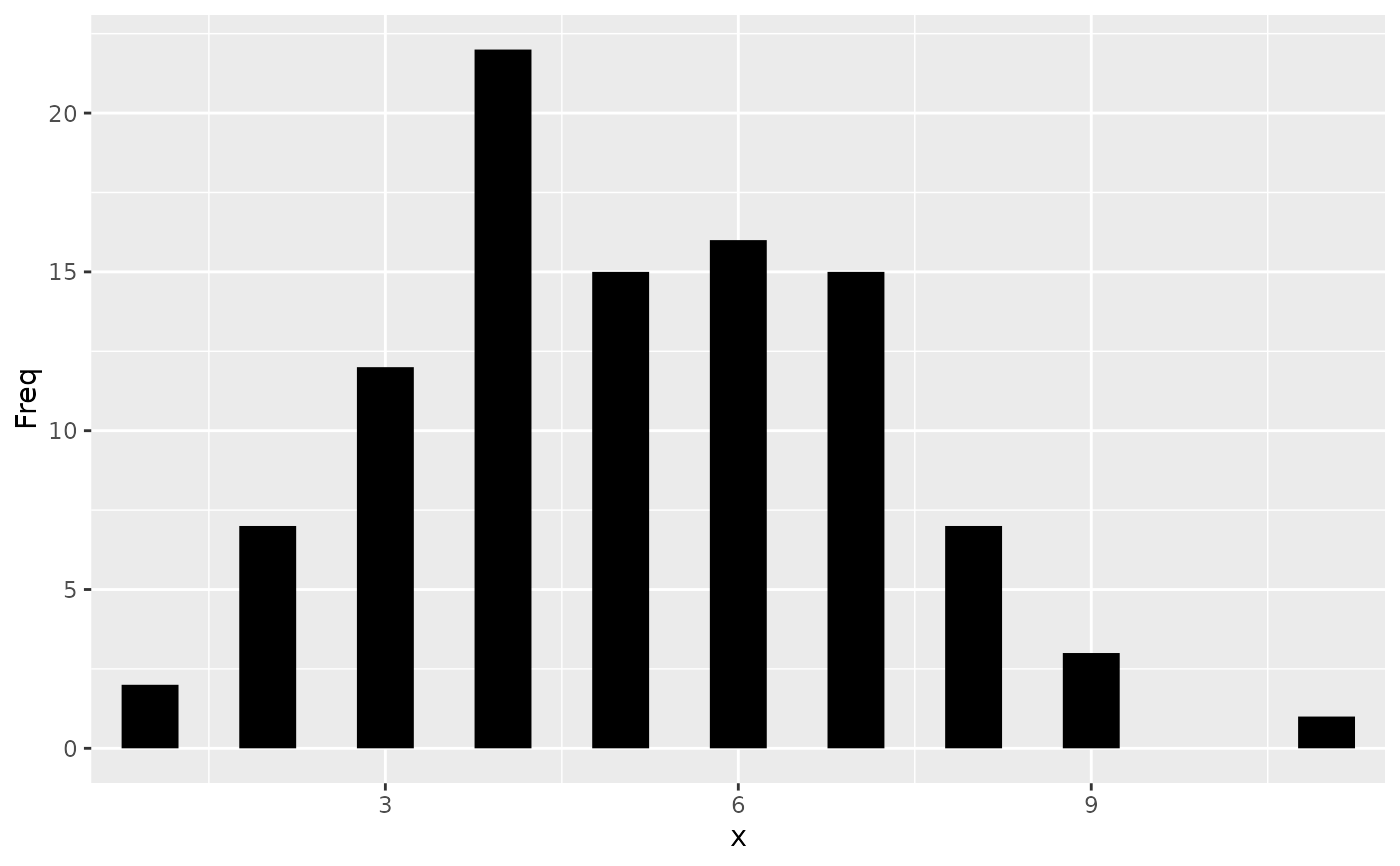The following aesthetics can be used to specify the position of elements: x, y, xmin, xmax, ymin, ymax, xend, yend.

## Details

x and y define the locations of points or of positions along a line or path.

x, y and xend, yend define the starting and ending points of segment and curve geometries.

xmin, xmax, ymin and ymax can be used to specify the position of annotations and to represent rectangular areas.

• Geoms that commonly use these aesthetics: geom_crossbar(), geom_curve(), geom_errorbar(), geom_line(), geom_linerange(), geom_path(), geom_point(), geom_pointrange(), geom_rect(), geom_segment()

• See also annotate() for placing annotations.

## Examples


# Generate data: means and standard errors of means for prices
# for each type of cut
dmod <- lm(price ~ cut, data = diamonds)
cut <- unique(diamonds$cut) cuts_df <- data.frame( cut, predict(dmod, data.frame(cut), se = TRUE)[c("fit", "se.fit")] ) ggplot(cuts_df) + aes( x = cut, y = fit, ymin = fit - se.fit, ymax = fit + se.fit, colour = cut ) + geom_pointrange()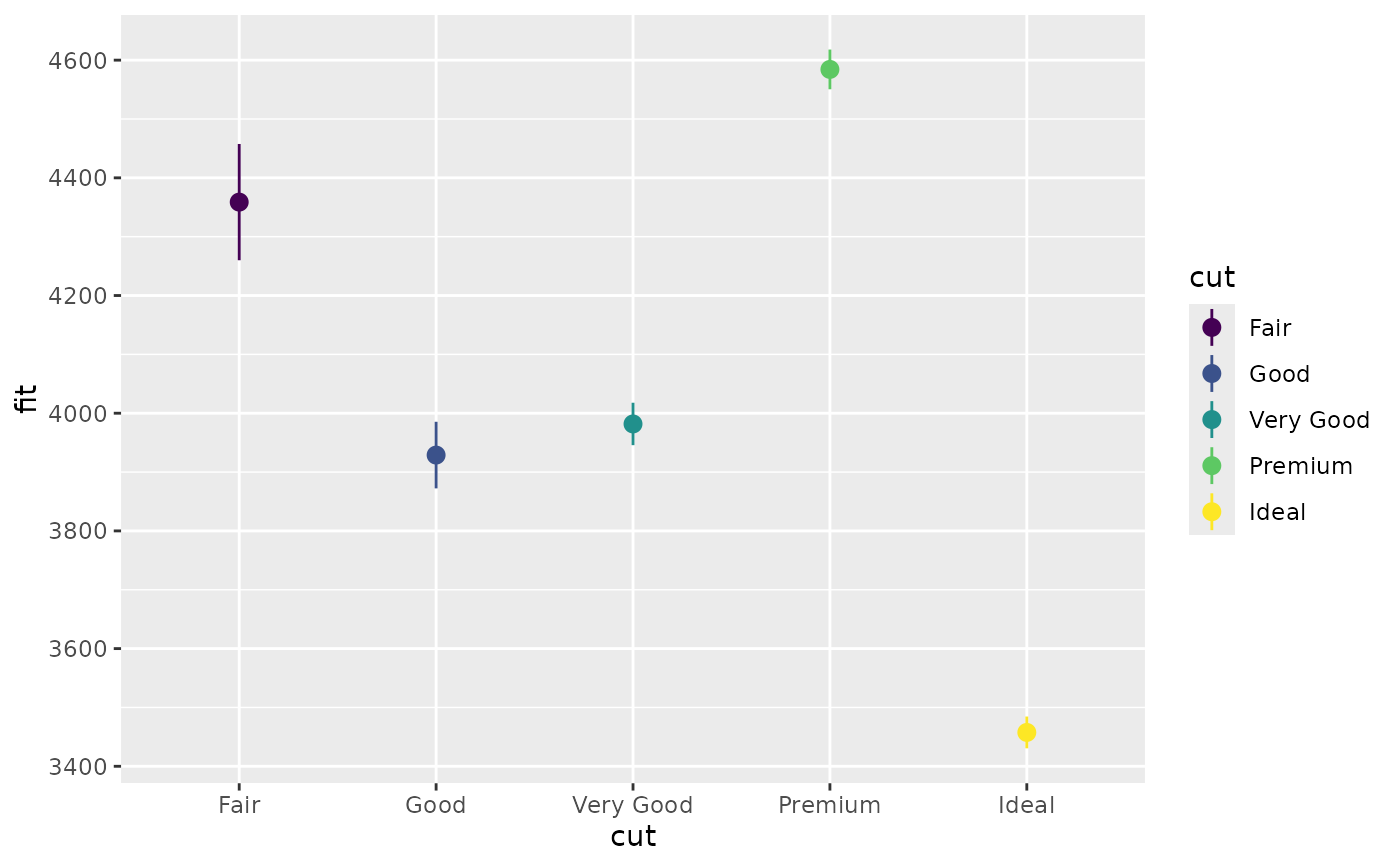# Using annotate p <- ggplot(mtcars, aes(x = wt, y = mpg)) + geom_point() p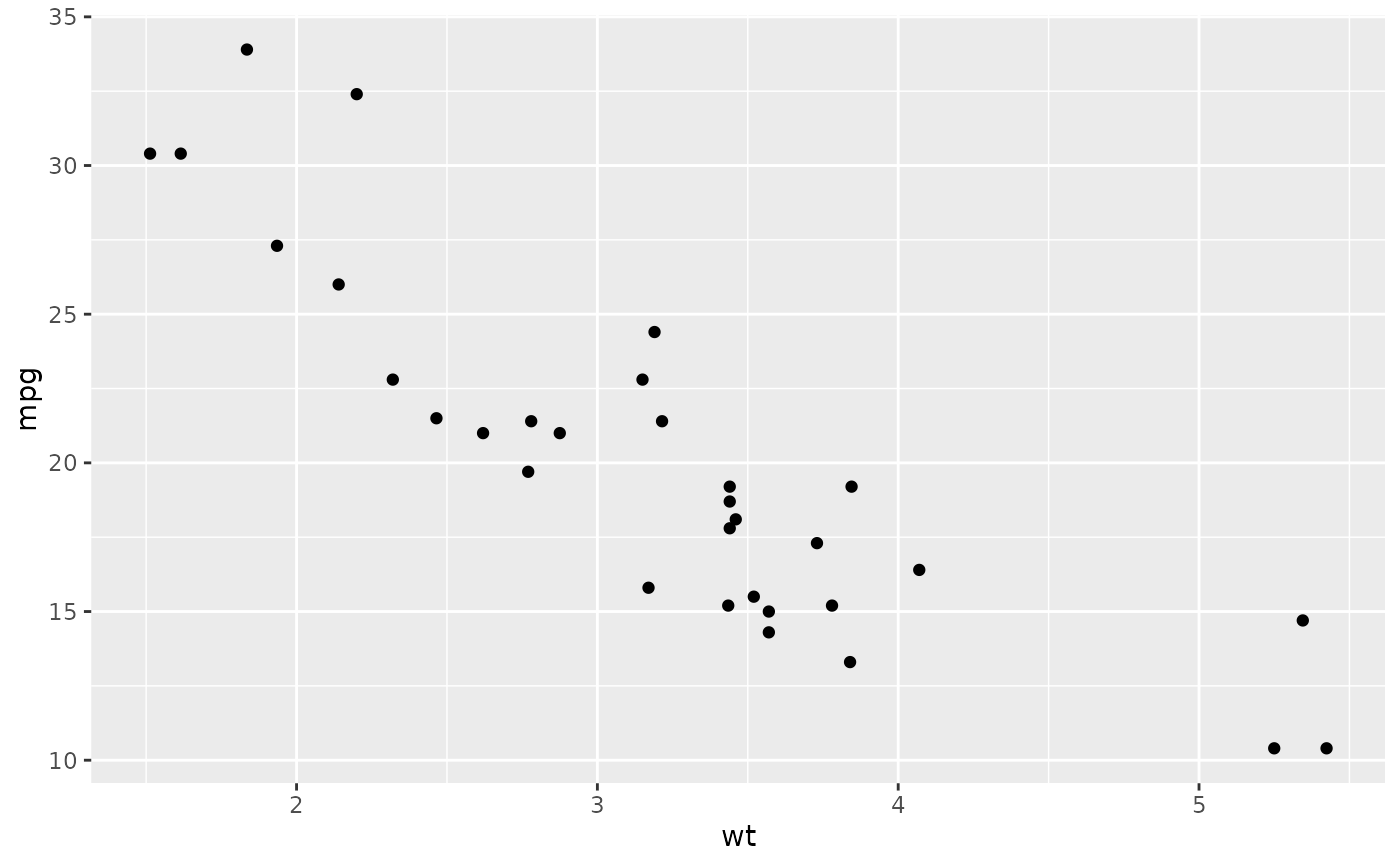p + annotate( "rect", xmin = 2, xmax = 3.5, ymin = 2, ymax = 25, fill = "dark grey", alpha = .5 )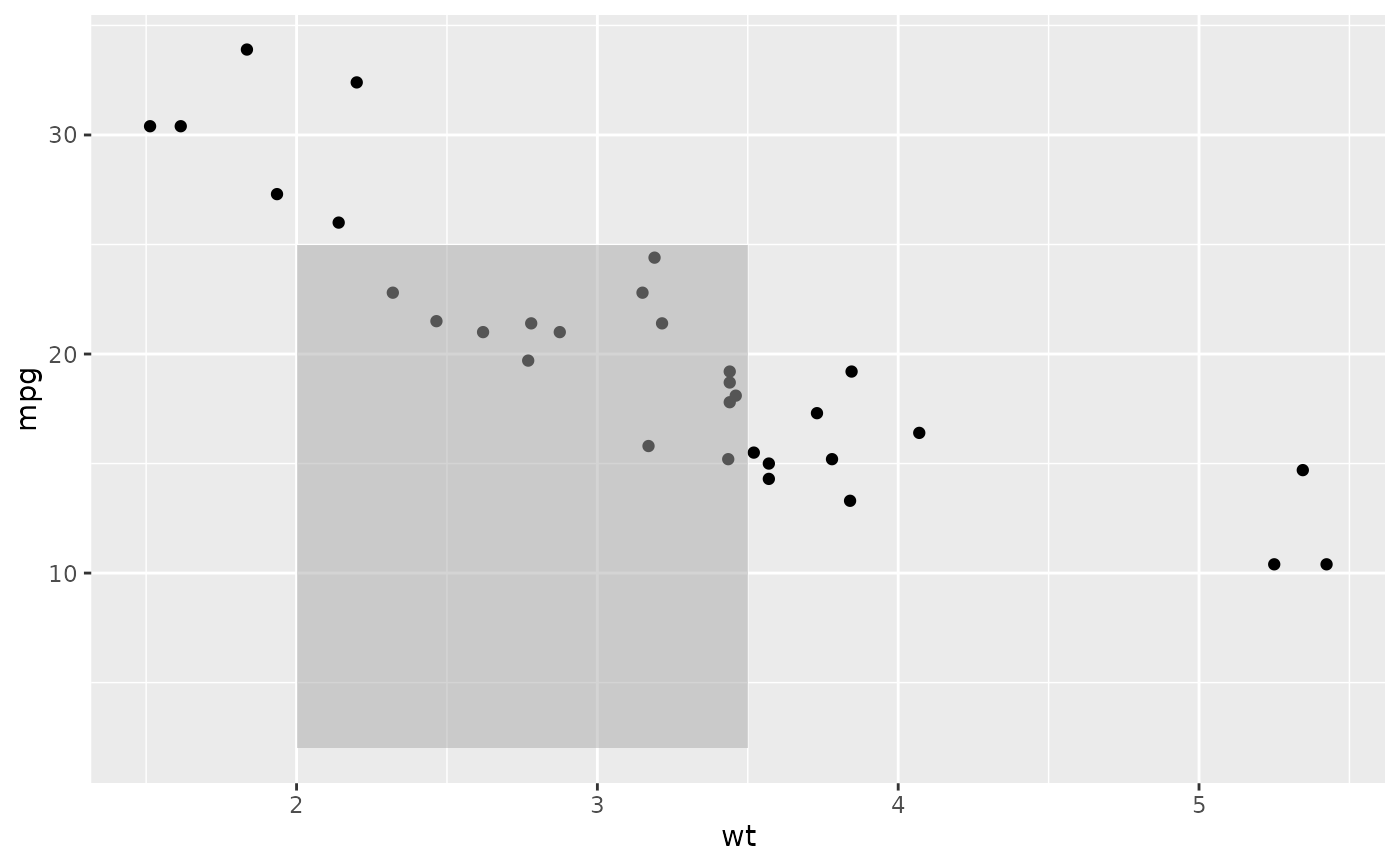# Geom_segment examples p + geom_segment( aes(x = 2, y = 15, xend = 2, yend = 25), arrow = arrow(length = unit(0.5, "cm")) )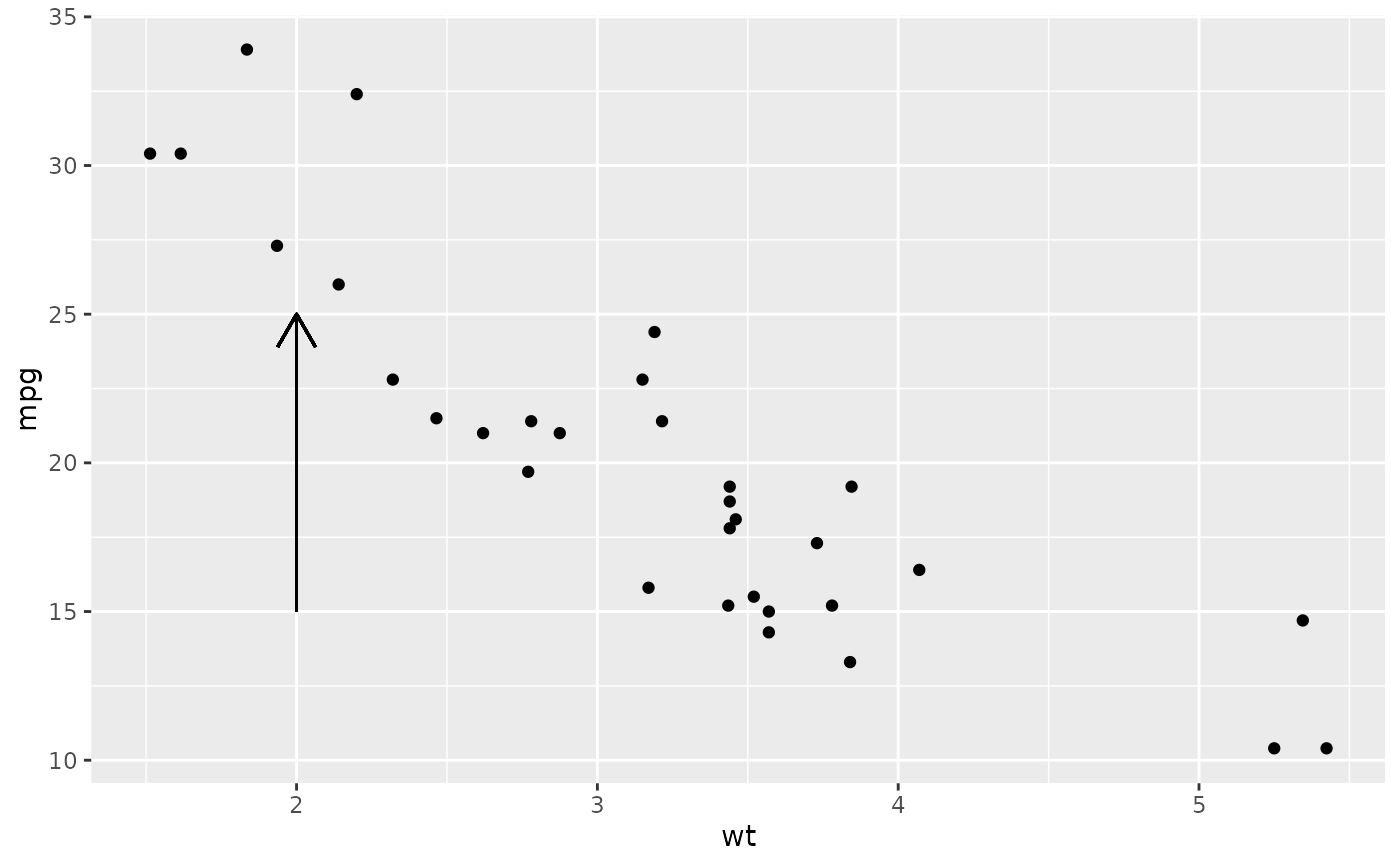p + geom_segment( aes(x = 2, y = 15, xend = 3, yend = 15), arrow = arrow(length = unit(0.5, "cm")) )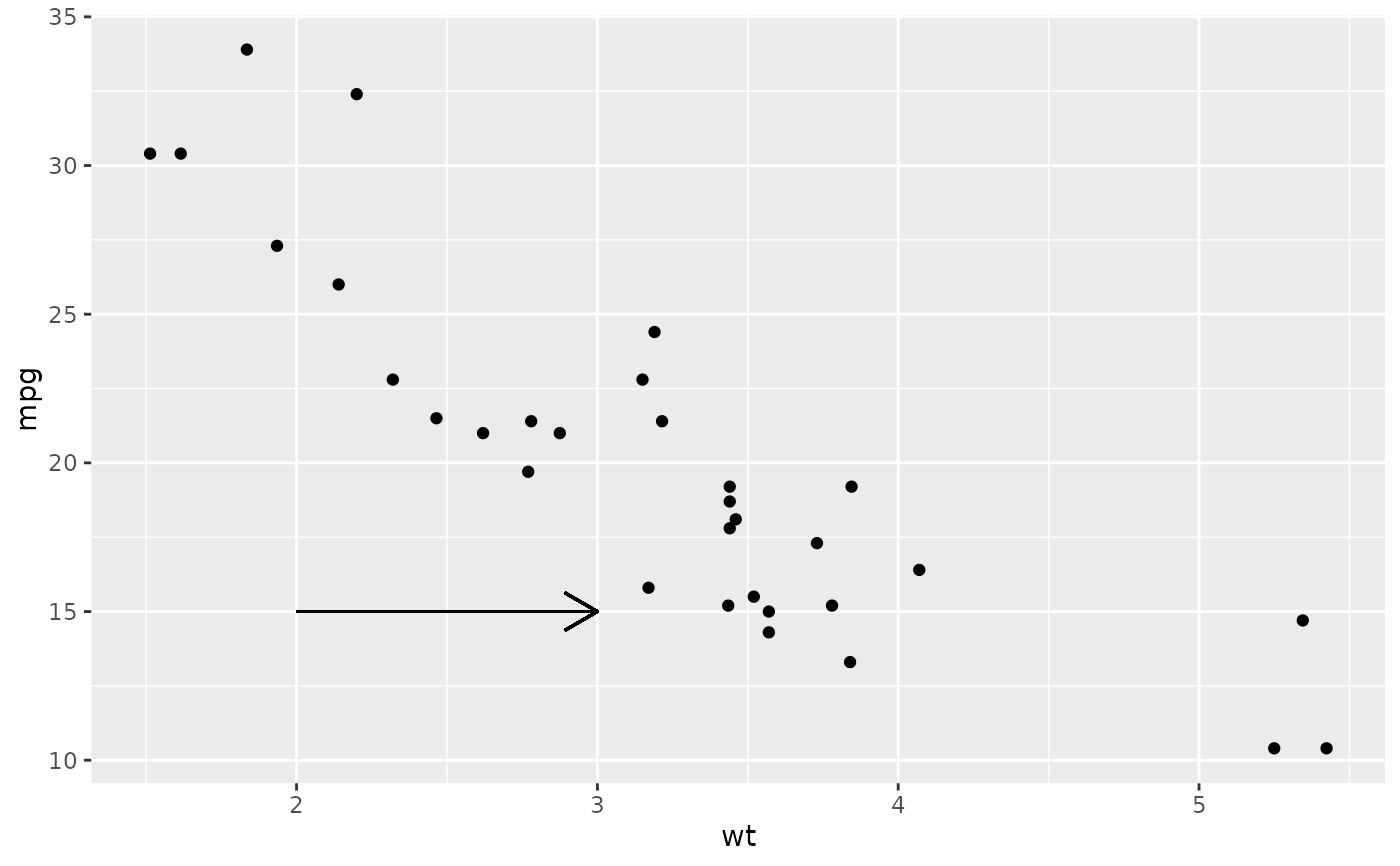p + geom_segment( aes(x = 5, y = 30, xend = 3.5, yend = 25), arrow = arrow(length = unit(0.5, "cm")) )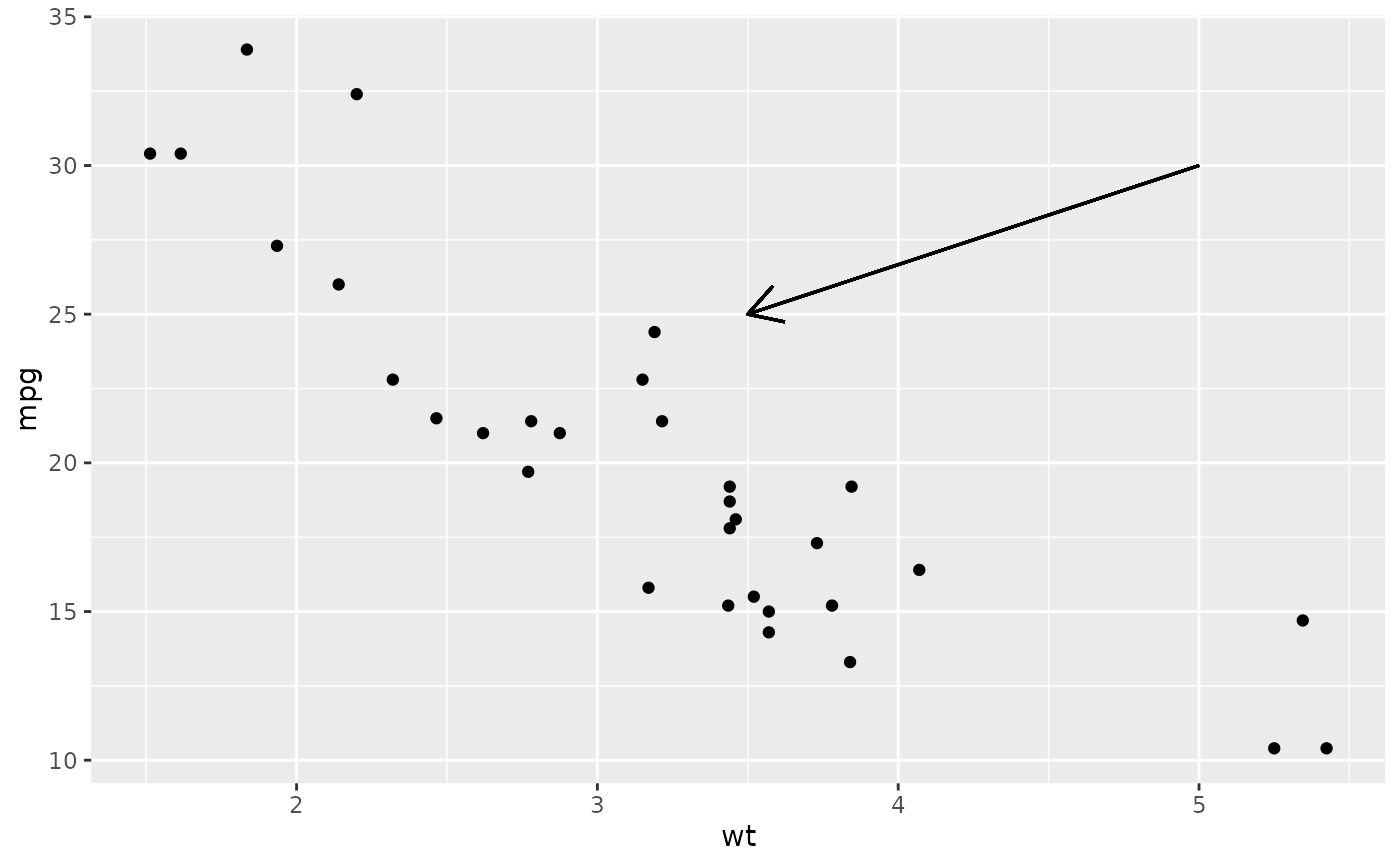# You can also use geom_segment() to recreate plot(type = "h") # from base R: counts <- as.data.frame(table(x = rpois(100, 5))) counts$x <- as.numeric(as.character(counts\$x))
with(counts, plot(x, Freq, type = "h", lwd = 10))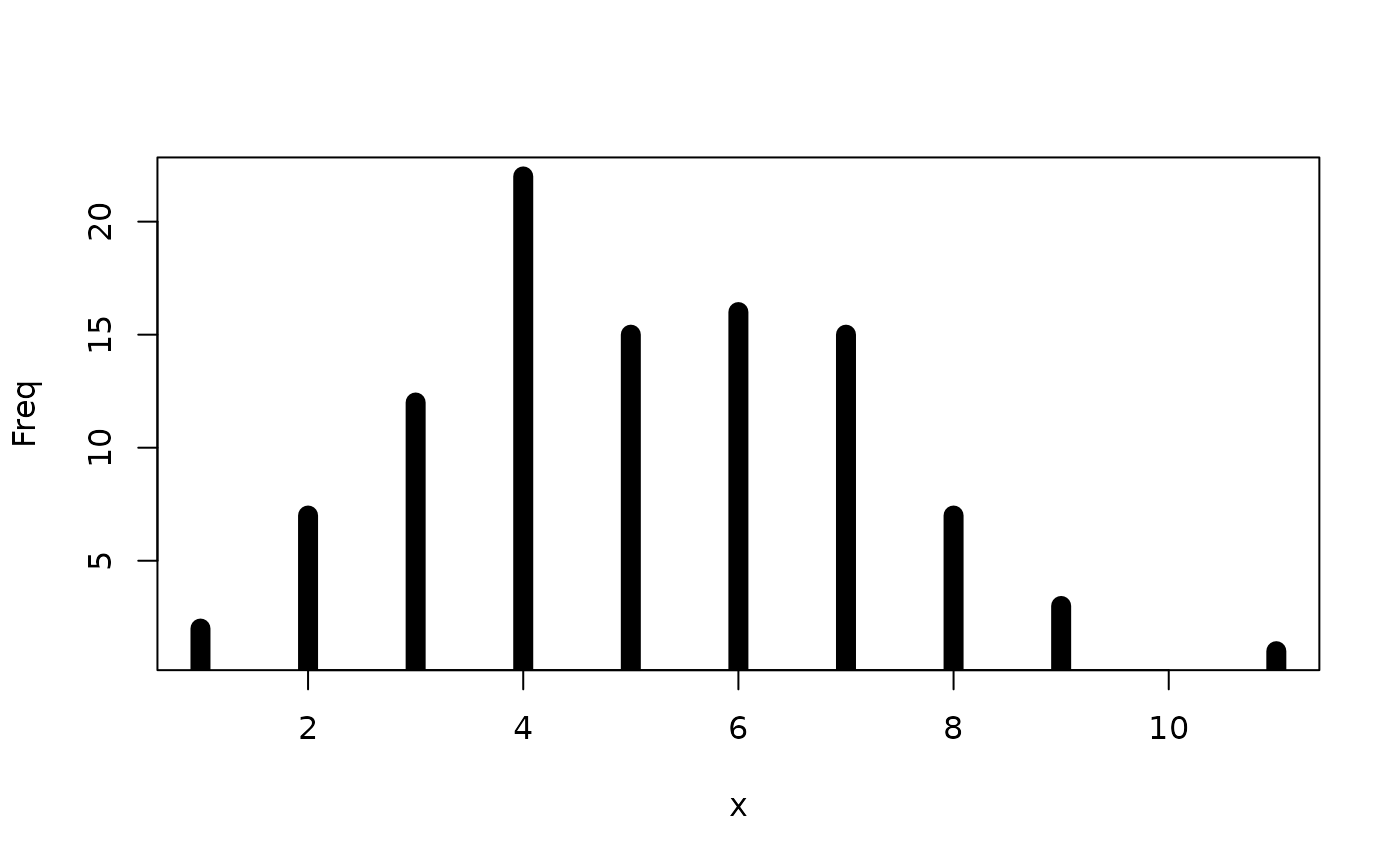ggplot(counts, aes(x = x, y = Freq)) +
geom_segment(aes(yend = 0, xend = x), size = 10)